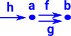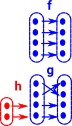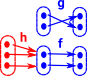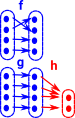# Maths - Category Theory - Equaliser and Coequaliser

### Equaliser

In set an injective function defines a subset relationship. In category theory this can be generalised to a monomorphism (monic).if h•f=h•g then f=g (h on left cancels out if it is monic)

### Coequaliser

In set an surjective function defines an equivalence relationship. In category theory this can be generalised to a epimorphism (epi).if f•h=g•h then f=g (h on right cancels out if it is epi)

### Injective and Surjective Functions in Set Theory

We are not really supposed to look inside sets in category theory but I find it helps me to get some intuition.

### Injective Functions

In set an injective function defines a subset relationship. In category theory this can be generalised to a monomorphism (monic).

If the injective function is after the pair then:

h•f=h•g does imply that f=g.### Equaliser

If the injective function is before the pair then:

f•h=g•h does not necessarily imply that f=g.

However an injective function before the pair can form an equaliser, this selects the elements where 'f' and 'g' agree.### Surjective Functions

In set an surjective function defines an equivalence relationship. In category theory this can be generalised to a epimorphism (epi).

If the surjective function is before the pair then:

f•h=g•h does imply that f=g.### Co-equaliser

If the surjective function is after the pair then:

h•f=h•g does not necessarily imply that f=g.

However an surjective function after the pair can form a co-equaliser, merges the elements where 'f' and 'g' do not agree and so the end-to-end function commutes.## Next

This page describes how equalisers can be generalised to limits and coequalisers can be generalised to colimits.